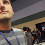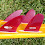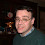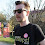## Tuesday, December 14, 2010

### Mathematical Question

A question to my knowledgeable readers: what's the correct way to represent a range of negative numbers? That is, if I have a table in which getting -1 through -2 yields one result and -3 through -5 yields another, how do I represent the ranges? I ask because it doesn't seem right that I should use "-1 - -2" and "-3 - -5." Do I use parentheses or brackets or some other way to separate the negative numbers from the dash between them?

Any help here would be appreciated. Thanks!

1.2.I would use parentheses.

3.In Excel, a range of cells is shown by putting a colon between the start and end cells, so your example would be show as -1:-2 .

4.-1 to -2 would be the correct way to present this from an editorial standpoint, and if you have both positive and negative ranges in one text, I would adjust the positive ranges to be the same format, e.g. 3 to 5.

5.Mathematically speaking, if you want to represent a closed set on a number line (i.e. you want to include the end points), then you would use brackets. An open set uses parentheses. You can also have half-open (or half closed if you are a pessimist) sets.

So [-5, -1] means the set of numbers x such that -5 <= x <= -1.

And (0, 5] means the set of numbers x such that 0 < x <= 5.

This notation is fairly specific to sets, however. I'm not sure how well it would play out in a table.

6.There is, at least in theory, a difference between an en-dash (used to mark a range) and a minus sign. So −3 to −1 might be represented "−3 – −1". But it's not much of an improvement. (And in the font my web browser happens to render comments here in, it's not visible.)

(I will note that the hyphen on your keyboard is likely neither an en-dash or a minus sign. The anal retentive among us appreciate using a proper minus sign in print. You can identify it because it's the same width as the plus sign.)

7.Myself, I'd go belts and suspenders: embrace each value parenthetically, and use the word to.

(-5) to (-3) Kumquat
(-2) to (-1) Pomegranate
0 Papaya
1 to 2 Apple
3 to 5 Banana

8.The Excel-style colon is the most elegant if used consistently for positive ranges as well. However, it's likely better to format it as a table in a printed text.

Parentheses work, but can make formulas look very odd when giving negative value examples.

9.I personally think you should use parentheses. Assuming the numerical value is the end result of a die roll (say d10 for argument's sake), I would represent it as follows. Note that this is my view as a consumer of roleplaying products and indicates my preferred mode based on readability, and is not based on any specific mathematical notation or rationale..

(-1) - (-2) entry
(-3) - (-5) entry
(-6) - (-8) entry
(-9) - (-10) entry

or something of the sort. If it were a positive range one would be used to seeing 1 - 2, 3 - 5, 6 - 8 (or whatever), and this seems consistent aesthetically.

10.an endash means through
so -1–-3 means -1 through -3

11.Alan, above, had the good suggestion from math, but it's a bit subtle for use when swilling beer and swinging swords. In programming, we'd often say: " -2 ... -1" and "-5 ... -3"

12.I've seen mathematics texts represent sequences using ellipses, for example -10, -9, ..., -1

Usually at least two leading numbers are listed, to make the pattern of repetition clear.

13.Regardless of delimiters, it makes more sense to me to write -5 to -2 rather than -2 to -5. That is, -5<-2 so we'd say -5 to -2, like we would say 3 to 5, ordered from left to right on the number line.

14.Personally, I would use the word "to" as in, "-5 to -2".

The question is not strictly a mathematical one; the question is more how you would best visually express the range in your particular game-table context. For that it's best to look at how some other games do it, e.g.:

- 1E Unearthed Arcana p. 15 (Cavaliers with negative XP) uses a large dash in the middle, with italicized numbers to set them off.
- 1E Unearthed Arcana 45 (Magic-users with negative XP for purpose of cantrips) uses the word "to" (although that's within text, not a table).
- 3E Player's Handbook p. 62 (example door DCs) uses the word "to" (even for positive numbers, no negatives included).

Perhaps others can dig up more examples. Again, I would use "to".

15.as was said mathematically
[-2, -1]
[-5, -3]
[-10, -6]
its the best way to do it

but I think that the
-2 to -1
-5 to -3
-10 to -6
is more clear and would be consistent with
1 to 2
3 to 5
and so on

16.I’m a typographer who encounter this problem from time to time, and I have to agree with those suggesting you use “to” instead of the en dash (which, incidentally, is the EXACT same character used as a minus sign).

17.I have found that when writing examples for 12 to 13 year old kids (I work with seventh graders), using a written phrase such as, "-5 to -2" to be the easiest understood.

I also try to (as mentioned above) to write it from the most negative as the first number listed and the larger number as the second. This is especially true if having positive results on the same table.

18.I was going to be cute and supply some unrelated mathematical equation here (like Schrodinger's Uncertainty Principle), but these blog comments don't handle the symbols very well... :P

I would say that the simplest answer is the best (as previously stated), "-1 to -3" or whatever range you require.